Function Repository Resource:

# OsculatingCircleProperties

Compute the defining properties of the osculating circle for a curve at a point

Contributed by: Wolfram|Alpha Math Team
 ResourceFunction["OsculatingCircleProperties"][f,{x,a}, y] gives the osculating circle of the curve f at the point x=a with respect to the variables x and y. ResourceFunction["OsculatingCircleProperties"][f,{x,a},{y,b}] gives the osculating circle of f at the point (a,b) with respect to the variables x and y. ResourceFunction["OsculatingCircleProperties"][{f[t],g[t]},{t,t0},x,y] gives the osculating circle to the parameterized curve at the point t0. ResourceFunction["OsculatingCircleProperties"][…,type] gives the property type of the osculating circle.

## Details

The property type can be All, "Radius", "Curvature", "Center", "Equation", "Function", "Circle", "Normal" and "Plot".
If f is an expression in ResourceFunction["OsculatingCircleProperties"][f,{x,a},y], the implicit curve is taken to be y==f.

## Examples

### Basic Examples (3)

Return the osculating circle for a given expression:

 In:=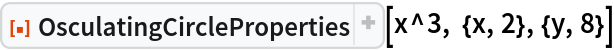Out=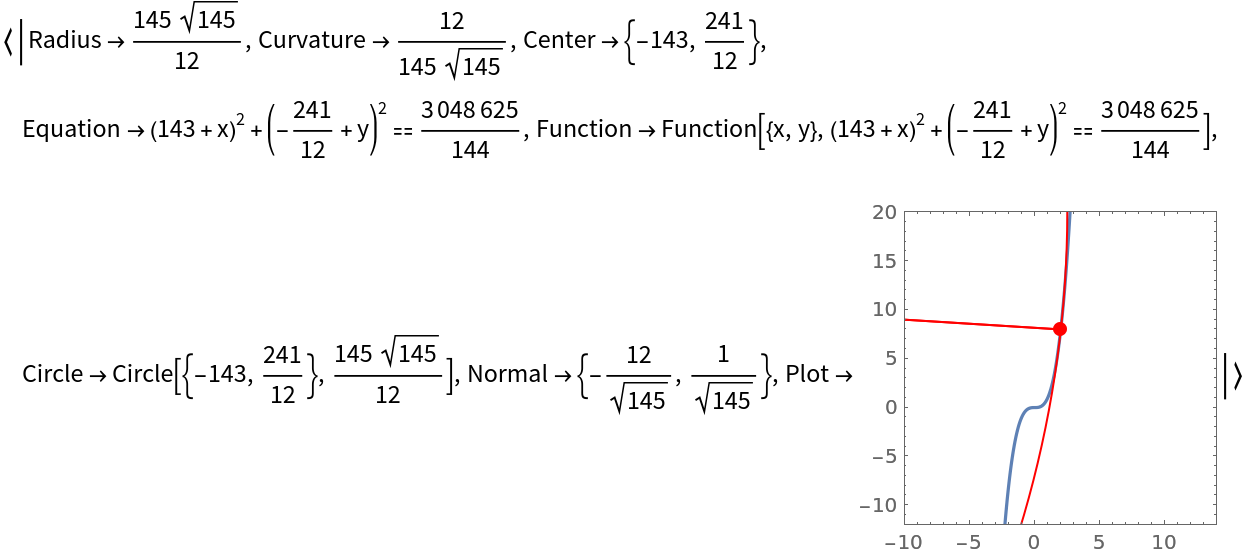Return the osculating circle for a parametrically defined curve:

 In:=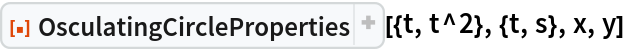Out=Return the osculating circle for an implicitly defined curve:

 In:=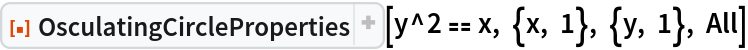Out=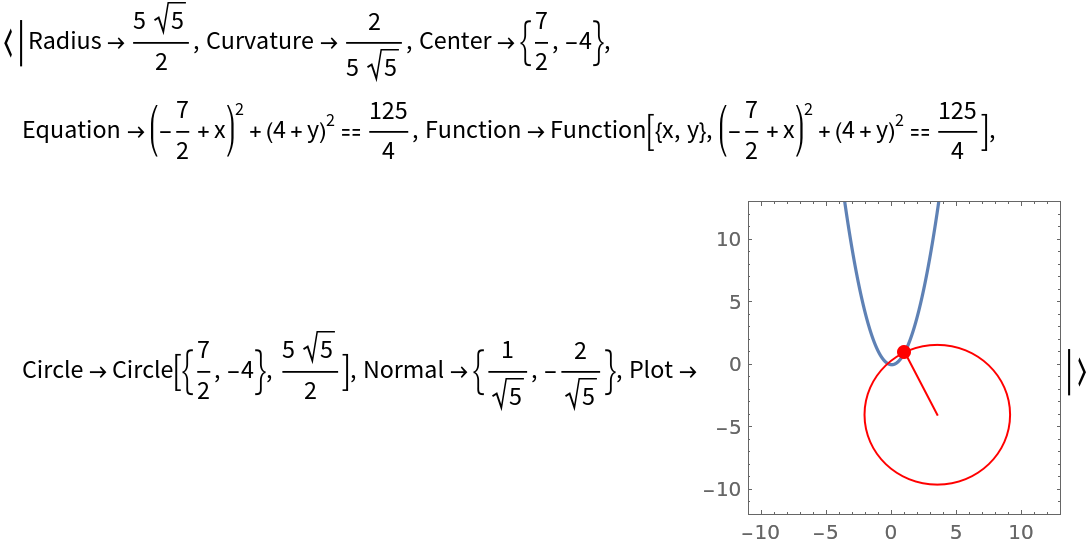### Applications (4)

Return the osculating circle:

 In:=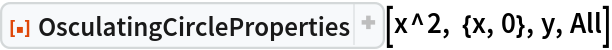Out=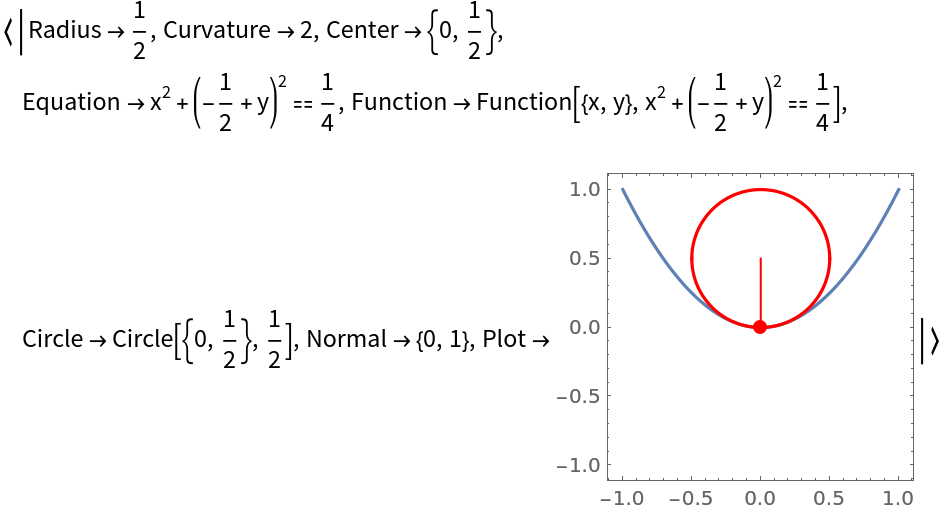Return only the circle as a Graphics primitive:

 In:=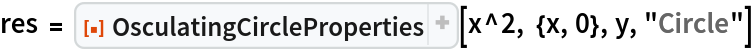Out=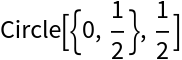Plot the osculating circle and expression:

 In:=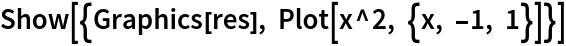Out=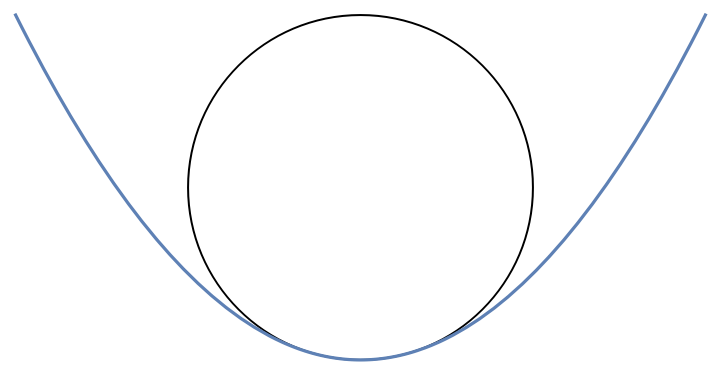Or view the plot directly:

 In:=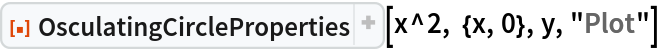Out=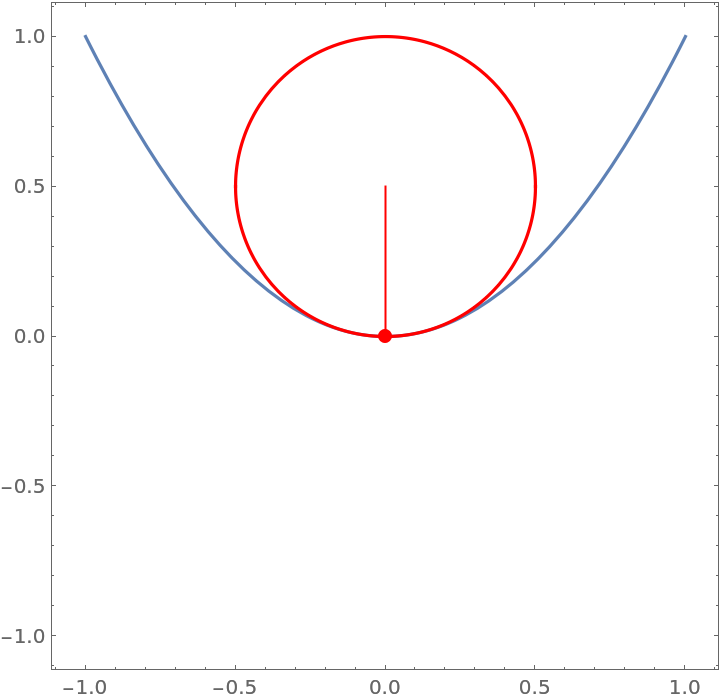### Possible Issues (2)

OsculatingCircleProperties returns unevaluated if the curvature does not exist at a given point: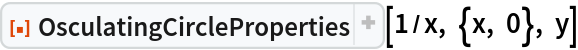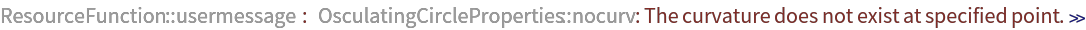Out=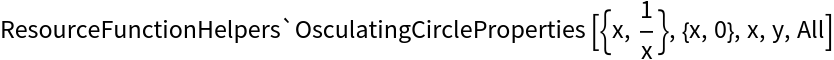OsculatingCircleProperties returns unevaluated if the gradient is zero: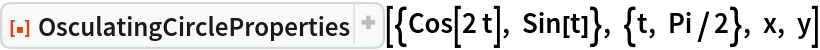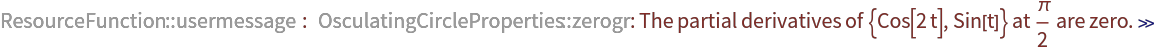Out=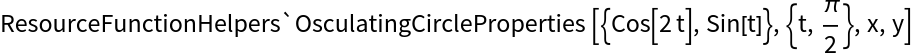## Publisher

Wolfram|Alpha Math Team

## Version History

• 2.0.0 – 23 March 2023
• 1.0.1 – 04 March 2021
• 1.0.0 – 31 March 2020

## Author Notes

To view the full source code for OsculatingCircleProperties, evaluate the following:

 In:=